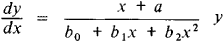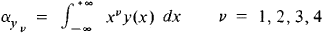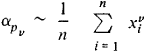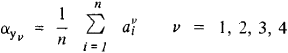# Pearson Curves

The following article is from The Great Soviet Encyclopedia (1979). It might be outdated or ideologically biased.

## Pearson Curves

a family of distribution curves—that is, curves y = y(x) representing the dependence on x of the probability density—that satisfy the differential equationwhere a, b0, b1, and b2 are real numbers. Pearson curves are classified into 12 types, depending on the values of the parameters a, b0, b1, and b2 and on the range of x. Examples of Pearson curves are the normal distribution, Student’s distribution, and the x2 distribution.

Any Pearson curve y (x) is uniquely determined by its first four moments:Pearson curves sometimes are used, on the basis of this property, in mathematical statistics for approximate representation of an unknown density p(x). For example, let there be a large series of independent observations x1, x2, …, xn of a random variable x with an unknown probability density p(x). Using the method of moments, we assume thatand for an approximate representation of p(x) we select a Pearson curve y(x) such thatPearson curves were first used to construct empirical densities by the English mathematician K. Pearson in 1894.

### REFERENCE

Kendall, M., and A. Stuart. Teoriia raspredelenii. Moscow, 1966. (Translated from English.)
The Great Soviet Encyclopedia, 3rd Edition (1970-1979). © 2010 The Gale Group, Inc. All rights reserved.
Mentioned in ?
References in periodicals archive ?
S., 1963, "Table of Percentage Points of Pearson Curves, for given [square root of [beta].sub.1] and [[beta].sub.2], Expressed in Standard Measure." Biometrika, 50:459-98.
In order to construct the control chart for costs, it was assumed that toal monthly log-costs were distributed as a Pearson curve. Under this assumption, the methods of Bowman and Shenton (1979) can be used to determine the control lines for charts of monthly total costs.

Site: Follow: Share:
Open / Close